# B.3 HEISENBERG Picture

In the HEISENBERG representation state vectors are defined as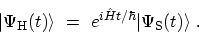(B.10)

Its time derivative may be combined with (B.3) to yield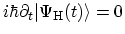, which shows that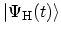is time-independent. Since an arbitrary matrix element in the SCHRÖDINGER picture can be written as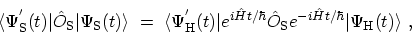(B.11)

a general operator in the HEISENBERG picture is given by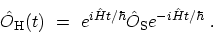(B.12)

Equation (B.12) can be rewritten in terms of the interaction picture operators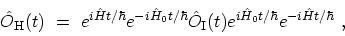(B.13)

or in terms of the operator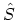derived in the next section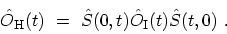(B.14)

M. Pourfath: Numerical Study of Quantum Transport in Carbon Nanotube-Based Transistors